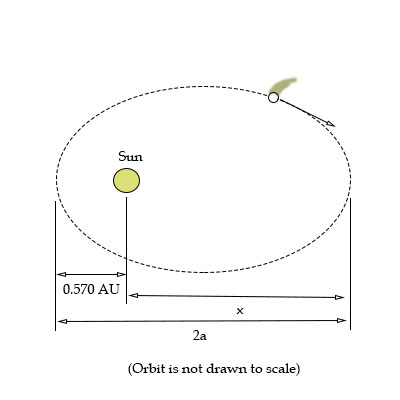# A comet (see figure below) approaches the Sun to within 0.570 \ AU, and its orbital periodis 90.6...

## Question:

A comet (see figure below) approaches the Sun to within {eq}0.570 \ AU {/eq}, and its orbital periodis {eq}90.6 {/eq} years. ({eq}AU {/eq} is the symbol for astronomical unit, where {eq}1 \ AU = 1.50\times 10^{11} \ m {/eq} is the mean Earth Sun distance.) How far from the Sun will the comet travel before it starts its return journey?## Kepler's Third Law

Kepler's third law depicts the relation between the time period of the planet or a massive body orbiting around the sun and its distance from the sun. It tells that the square of the orbital time period of a planet of a body revolving around the sun is directly proportional to the cube of the semi-major axis of the orbit.

Become a Study.com member to unlock this answer! Create your account

Given data:

• The orbital period of the comet is: {eq}T = 90.6\,{\rm{years}} {/eq}

Write the expression for the semi major axis of the elliptical...